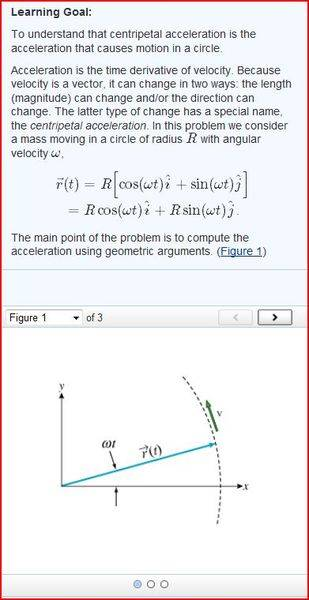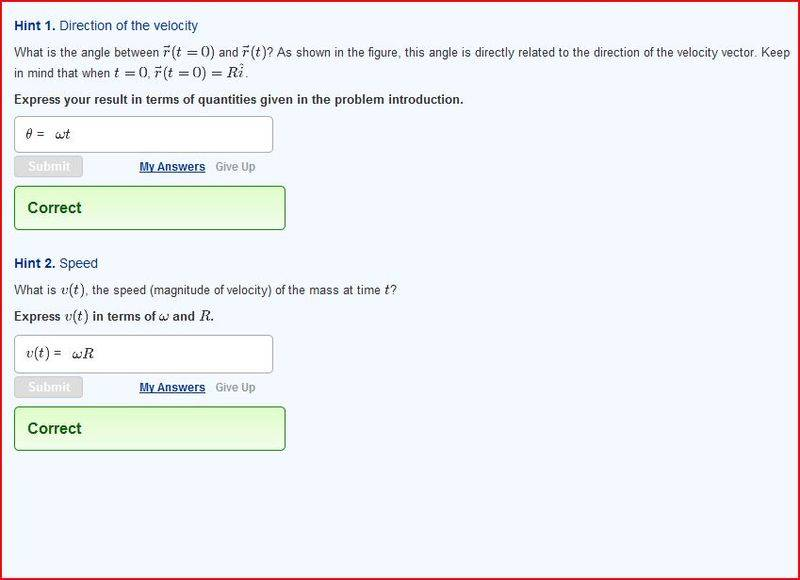# Rotational motion

R

## Homework StatementA
What is the velocity of the mass at a time t? You can work this out geometrically with the help of the hints, or by differentiating the expression for r⃗ (t) given in the introduction. (Figure 2)
Express this velocity in terms of R, ω, t, and the unit vectors i^ and j^.

B
Assume that the mass has been moving along its circular path for some time. You start timing its motion with a stopwatch when it crosses the positive x axis, an instant that corresponds to t=0. [Notice that when t=0, r⃗ (t=0)=Ri^.] For the remainder of this problem, assume that the time t is measured from the moment you start timing the motion. Then the time − t refers to the moment a time t before you start your stopwatch.

What is the velocity of the mass at a time − t?
Express this velocity in terms of R, ω, t, and the unit vectors i^ and j^.

C
What is the average acceleration of the mass during the time interval from − t to t? (Figure 3)
Express this acceleration in terms of R, ω, t, and the unit vectors i^ and j^.

2. Homework Equations

V=rω
a=rα
Rotational kinematics equations

## The Attempt at a Solution

For A:So from my understanding the radius will vary with time and ω but I do not see the relevance of that.

If V=rω
and the r given is R(cos(ωt){i}+sin(ωt){j}) then shouldn't the v be
R(cos(ωt){i}+sin(ωt){j}) * ω ?

I do not understand this question, what does differentiation have to do with this? That would just give me how it is changing, not v

Velocity is the change in position over the change in time. This is a rate of change. A derivative is the instantaneous rate of change at a particular point. Acceleration is the change in velocity over change in time. When an objet is travelling in a circular path, the direction of its velocity vector is changing, therefore the object is experiencing an acceleration. The question is guiding you towards determining the acceleration of an object undergoing circular motion.

so then I just take the derivative of the radius? but is my thinking wrong?
so does

v=rω
v= d/t

rω=d/t ?

And for acceleration do I use radial acceleration v2/R or linear acceleration v/t?

and If I use linear acceleration which v do I use?